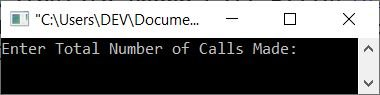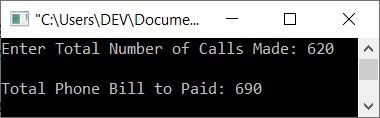# C++ program to calculate telephone call bills

This article provides some programs in C++ that find and print the bill amount based on the telephone calls made. The number of telephone calls must be entered by the user at run-time. Here is the list of programs this article deals with:

The telephone call bills are charged in this way:

• The first 150 calls are free.
• The next 250 calls (151-400 minutes) are charged at the rate of 1/minute.
• And all calls after 400 minutes are charged at the rate of 2/minute.

For example, if the customer made a total call of 620 minutes, The phone call bill charge is then calculated as follows:

```620 calls = 150 (Free) + 250 (at 1/minute) + 220 (at 2/minute)
= 0 + (250*1) + (220*2)
= 250 + 440
= 690```

As a result, 690 is the phone call bill that will be charged after speaking for 620 minutes.

## Calculate phone bills without using the function in C++

The question is: write a program in C++ that calculates and prints the phone bills that have to be paid based on the total calls entered by the user at run-time. The program given below is the answer to this question:

```#include<iostream>

using namespace std;
int main()
{
int ncall, temp, charge;
cout<<"Enter Total Number of Calls Made: ";
cin>>ncall;
if(ncall<=150)
cout<<"\nNo charge!";
else
{
ncall = ncall - 150;
if(temp<=250)
charge = temp*1;
else
{
temp = ncall-250;
charge = 250 + (temp*2);
}
cout<<"\nTotal Phone Bill to Paid: "<<charge;
}
cout<<endl;
return 0;
}```

Here is the initial output produced by the above C++ program on calculating phone call bills based on the calls made:Now type or enter the total number of calls made to find and print the charge that has to be paid. Here's a sample run with 620 as the total number of calls (in minutes) made:## Calculate telephone call bills using a user-defined function in C++

This program does the same job as the previous program. But the only difference is that this program is created using a user-defined function named phone_call() that takes the number of calls made as its argument and returns the bill amount that has to be paid based on the calls made.

```#include<iostream>

using namespace std;
int phone_call(int);
int main()
{
int calls, amount;
cout<<"Enter Total Number of Calls Made: ";
cin>>calls;
amount = phone_call(calls);
cout<<"\nTotal Phone Bill to Paid: "<<amount;
cout<<endl;
return 0;
}
int phone_call(int ncall)
{
int temp, charge;
if(ncall<=150)
return 0;
else
{
ncall = ncall - 150;
if(temp<=250)
charge = temp*1;
else
{
temp = ncall-250;
charge = 250 + (temp*2);
}
return charge;
}
}```

This program does the same job as the previous program, with exactly the same-looking output as the previous program's output.

## Using classes and objects, compute telephone call bills in C++

This is the final program in this article, written in class. That is, a class named "CodesCracker" is created with two private variables, "temp" and "charge", and a public member function called "phone_call()". And using the object (cobj) of this class (as created inside main()), the member function phone_call() gets accessed.

```#include<iostream>

using namespace std;

class CodesCracker
{
private:
int temp, charge;
public:
int phone_call(int);
};
int CodesCracker::phone_call(int ncall)
{
if(ncall<=150)
return 0;
else
{
ncall = ncall - 150;
if(temp<=250)
charge = temp*1;
else
{
temp = ncall-250;
charge = 250 + (temp*2);
}
return charge;
}
}

int main()
{
int calls, amount;
CodesCracker cobj;

cout<<"Enter Total Number of Calls Made: ";
cin>>calls;

amount = cobj.phone_call(calls);
cout<<"\nTotal Phone Bill to Paid: "<<amount;
cout<<endl;
return 0;
}```

This program also does the same job as the very first one and produces similar output as that one. The only difference is its approach. That is, this program is created using classes and objects.

C++ Quiz

« Previous Program Next Program »